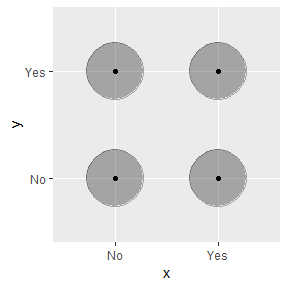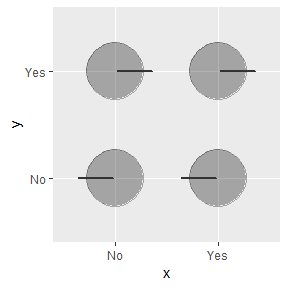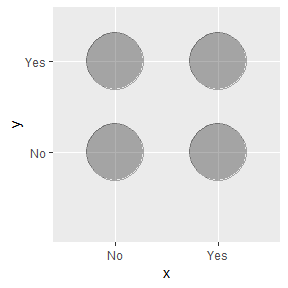# nonet_ensemble classification with nonet_plot

### nonet provides ensemble capabilities for classification problems.

Below example shows the step by step implementation of nonet_ensemble and nonet_plot functions in the context of classification. We have used Bank Note authentication data set to predict the output class variable using random forest and neural network models. Predictions from random forest model and neural network model are being used as inputs to the nonet_ensemble in the list form.

Let’s start:

library(caret)
## Loading required package: lattice
## Loading required package: ggplot2
library(ggplot2)
library(nonet)

#### Load the banknote_authentication dataset and explore it.

dataframe <- data.frame(banknote_authentication)
head(dataframe)
##   variance skewness curtosis  entropy class
## 1  3.62160   8.6661  -2.8073 -0.44699     0
## 2  4.54590   8.1674  -2.4586 -1.46210     0
## 3  3.86600  -2.6383   1.9242  0.10645     0
## 4  3.45660   9.5228  -4.0112 -3.59440     0
## 5  0.32924  -4.4552   4.5718 -0.98880     0
## 6  4.36840   9.6718  -3.9606 -3.16250     0

We can see above that class variable has int datatype, we need to convert it into factor so that classification models can be trained on that.

#### Converting datatype of class variable into factor which have two classes Yes and No.

dataframe$class <- as.factor(ifelse(dataframe$class >= 1, 'Yes', 'No'))
dataframe <- data.frame(dataframe)
head(dataframe)
##   variance skewness curtosis  entropy class
## 1  3.62160   8.6661  -2.8073 -0.44699    No
## 2  4.54590   8.1674  -2.4586 -1.46210    No
## 3  3.86600  -2.6383   1.9242  0.10645    No
## 4  3.45660   9.5228  -4.0112 -3.59440    No
## 5  0.32924  -4.4552   4.5718 -0.98880    No
## 6  4.36840   9.6718  -3.9606 -3.16250    No

index <- createDataPartition(dataframe$class, p=0.75, list=FALSE) trainSet <- dataframe[ index,] testSet <- dataframe[-index,] #### Feature selection using rfe in caret control <- rfeControl(functions = rfFuncs, method = "repeatedcv", repeats = 3, verbose = FALSE) outcomeName <- 'class' predictors <- c("variance", "skewness", "curtosis", "entropy") #### Model Training: Random forest banknote_rf <- train(trainSet[,predictors],trainSet[,outcomeName],method='rf') #### Model Training: neural network banknote_nnet <- train(trainSet[,predictors],trainSet[,outcomeName],method='nnet') Now we need to predict the outcome on testSet using the trained models #### Predictions on testSet in probabilities predictions_rf <- predict.train(object=banknote_rf,testSet[,predictors],type="prob") predictions_nnet <- predict.train(object=banknote_nnet,testSet[,predictors],type="prob") #### Predictions on testSet in raw form i.e in levels predictions_rf_raw <- predict.train(object=banknote_rf,testSet[,predictors],type="raw") predictions_nnet_raw <- predict.train(object=banknote_nnet,testSet[,predictors],type="raw") #### Create the stack of prediction probabilities for the class of Yes Stack_object <- list(predictions_rf$Yes, predictions_nnet$Yes) #### Applying naming to the Stack_object names(Stack_object) <- c("model_rf", "model_nnet") #### Convet list object into dataframe Stack_object_df <- data.frame(Stack_object) #### nonet_ensemble Now we need to apply the nonet_ensemble method by supplying list object and best model name as input. Note that We have not provided training or test outcome labels to compute the weights in the weighted average ensemble method, which is being used inside the none_ensemble. Thus it uses best models prediction to compute the weights in the weighted average ensemble. prediction_nonet_raw <- nonet_ensemble(Stack_object, "model_nnet") #### Convert probabilities into factor levels. prediction_nonet <- as.factor(ifelse(prediction_nonet_raw >= "0.5", "Yes", "No")) #### Evaluation Matrix: nonet Here Confusion matrix is being used to evaluate the performance of nonet, rf and nnet. nonet_eval <- confusionMatrix(prediction_nonet, testSet[,outcomeName]) nonet_eval_rf <- confusionMatrix(predictions_rf_raw,testSet[,outcomeName]) nonet_eval_nnet <- confusionMatrix(predictions_nnet_raw,testSet[,outcomeName]) nonet_eval_df <- data.frame(nonet_eval$table)
nonet_eval_rf_df <- data.frame(nonet_eval_rf$table) nonet_eval_nnet_df <- data.frame(nonet_eval_nnet$table)

#### Result Plotting: nonet_plot

Results can be plotted using the nonet_plot function. nonet_plot is being designed to provided different plot_type options to the user so that one can plot different visualization based on their needs.

##### nonet_plot for the result of nonet_ensemble models predictions
plot_first <- nonet_plot(nonet_eval_df$Prediction, nonet_eval_df$Reference, nonet_eval_df, plot_type = "point")
plot_first##### nonet_plot for the result of random forest model’s predictions
plot_second <- nonet_plot(nonet_eval_rf_df$Prediction, nonet_eval_rf_df$Reference, nonet_eval_rf_df, plot_type = "boxplot")
plot_second##### nonet_plot for the result of neural network model’s predictions
plot_third <- nonet_plot(nonet_eval_nnet_df$Prediction, nonet_eval_nnet_df$Reference, nonet_eval_nnet_df, plot_type = "density")
plot_third### Conclusion

Above it can be seen that nonet_ensemble and nonet_plot can serve in a way that one do not need to worry about the outcome variables labels to compute the weights of weighted average ensemble solution.